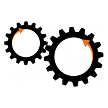# What's New for May: Programs and Multi-line Functions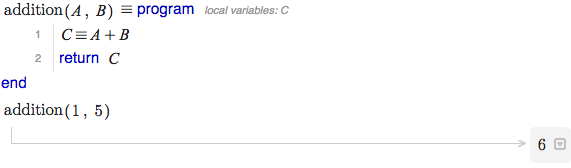A new month means new features at Swift Calcs, and today we are excited to announce the availability of multi-line functions, which we call programs, in Swift Calcs.

## Functions

Often times when performing an engineering calculation, we have create a set of steps to determine an answer based on one or more inputs. When we can simplify this down to a single line, we can use a function in Swift Calcs.

But what if we need more than one line? Swift Calcs has allowed conditional piece-wise functions since December, but any other multi-line functions or structures were not possible, until now.

## Introducing Programs

Multi-line functions in Swift Calcs are called programs. With a program, a set of Swift Calcs commands are pre-compiled before use, greatly speeding calculation time and allowing for complex calculations to be repeated for a number of inputs or settings.

Programs allow all normal Swift Calcs commands that set the value of variables or functions, including math mode, solvers, and other tools from the toolbox. Chain commands together just like in a worksheet, and return your value for later use.

## An Example

The easiest way to understand programs is by looking at an example. Consider finding the solution to a cubic equation in Swift Calcs: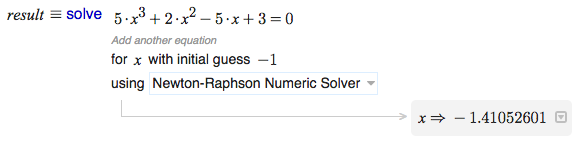What if we wanted to know the solution for a number of cubic equations with a differing cubic term coefficient? Before programs, we would have to copy the solver line multiple times, and update it each time for the value of the coefficient we want to solve for.

Programs provide another way. By using a program, we can wrap the solver into a function definition, and return the value: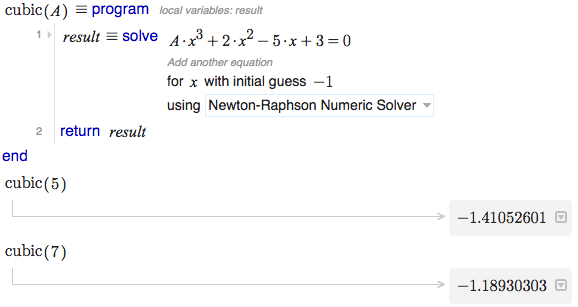Here, we have defined a program, cubic, with a single input, A. Inside the program, we utilize the solver, which depends on A, to find the solution near -1 to our cubic equation, and store it in a local variable, result. We finally use the return statement to return the value in result to the function.

On the next lines, we call the cubic program like we would any other function, and the solution is immediately returned. You can use programs anywhere like you would a function, even in plots...let's plot the cubic program for input values from 4 to 8: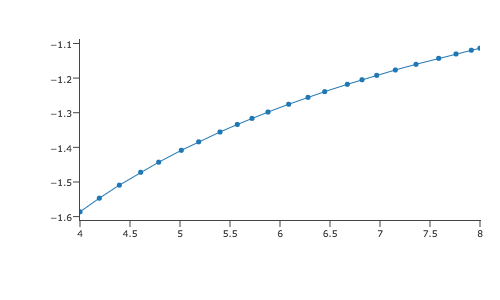Or use the solver to find the cubic coefficient that has a root at -1.35: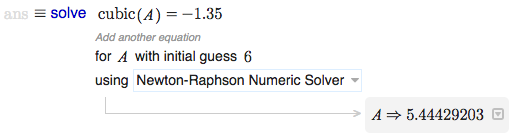Programs can have any number of inputs, and any number of internal lines, to produce a single output. Multiple outputs can be returned as an array. Flow control, including if statements and for loops, allow you to add complexity and logic to your programs. And like any other function or variables, whole programs can be included in to other worksheets using the include worksheet tool.

## Getting Started

To create a program, click and drag the program tool from the Programming section of the toolbox on to your worksheet. Alternatively, you can type program followed by a space on a new math-mode line.

Need more information? Check out the help article on programs and our new example on multivariate hypergemoetric distributions to see a complex program in action.